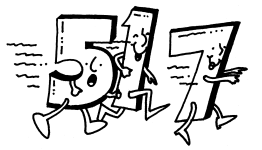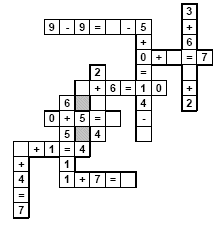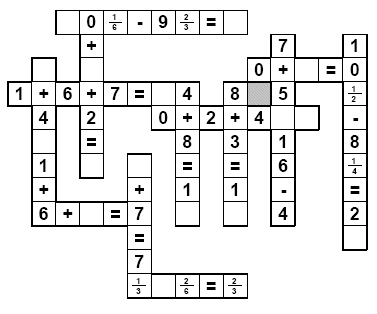Worksheets and No Prep Teaching Resources Runaway Math UnitThird GradeRunaway Math

Runaway Math Puzzles
Fifth and sixth grade runaway math puzzles
Runaway math puzzles with fractions

Create a custom Runaway Math Puzzle
Create a custom runaway math puzzle
Create a custom runaway math puzzle with missing equations
Create a custom runaway math puzzle where everything is missing

Runaway Math Puzzles: Third Grade - No FractionsAddition - Missing All the Numbers in Equations
One equation missing (only numbers)
Two equations missing (only numbers)
Three equations missing (only numbers)
One equation missing
Two equations missing
Three equations missing
Addition - Missing Numbers (smaller puzzle)
Three numbers missing
Five numbers missing
Seven numbers missing
Three numbers missing and all of the operations missing
Addition - Missing Numbers (larger puzzle)
Five numbers missing
Seven numbers missing
Nine numbers missing
Addition - Missing Pieces (may be a number or a symbol)
Three pieces missing
Five pieces missing
Seven pieces missing
Addition - Missing Pieces (may be a number or a symbol; larger puzzle)
Five pieces missing
Seven pieces missing
Nine pieces missing
20 % of puzzle missing (smaller puzzle)
40 % of puzzle missing (smaller puzzle)
20 % of puzzle missing
40 % of puzzle missing

Subtraction - Missing All the Numbers in Equations
One equation missing (only numbers)
Two equations missing (only numbers)
Three equations missing (only numbers)
Subtraction - Missing Entire Equations
One equation missing
Two equations missing
Three equations missing
Subtraction - Missing Numbers (smaller puzzle)
Three numbers missing
Five numbers missing
Seven numbers missing
Three numbers missing and all of the operations missing
Subtraction - Missing Numbers (larger puzzle)
Five numbers missing
Seven numbers missing
Nine numbers missing
Subtraction - Missing Pieces (may be a number or a symbol)
Three pieces missing
Five pieces missing
Seven pieces missing
Subtraction - Missing Pieces (may be a number or a symbol; larger puzzle)
Five pieces missing
Seven pieces missing
Nine pieces missing
Subtraction - Missing Pieces
20 % of puzzle missing (smaller puzzle)
40 % of puzzle missing (smaller puzzle)
20 % of puzzle missing
40 % of puzzle missing

Addition and Subtraction - Missing All the Numbers in Equations
One equation missing (only numbers)
Two equations missing (only numbers)
Three equations missing (only numbers)
Addition and Subtraction - Missing Entire Equations
One equation missing
Two equations missing
Three equations missing
Addition and Subtraction - Missing Numbers (smaller puzzle)
Three numbers missing
Five numbers missing
Seven numbers missing
Three numbers missing and all of the operations missing
Addition and Subtraction - Missing Numbers (larger puzzle)
Five numbers missing
Seven numbers missing
Nine numbers missing
Addition and Subtraction - Missing Pieces (may be a number or a symbol)
Three pieces missing
Five pieces missing
Seven pieces missing
Addition and Subtraction - Missing Pieces (may be a number or a symbol; larger puzzle)
Five pieces missing
Seven pieces missing
Nine pieces missing
Addition and Subtraction - Missing Pieces
20 % of puzzle missing (smaller puzzle)
40 % of puzzle missing (smaller puzzle)
20 % of puzzle missing
40 % of puzzle missing

Multiplication - complete the equation (no critical thinking)
Up to three equations with one missing number
All of the equations with one missing number
Multiplication - Two Missing Numbers from Equations
Two equations are each missing at least two numbers
All of the equations are each missing at least two numbers (not easy)
Multiplication - Missing All the Numbers in Equations
One equation missing (only numbers)
Two equations missing (only numbers)
Three equations missing (only numbers)
Multiplication - Missing Entire Equations
One equation missing
Two equations missing
Three equations missing
Multiplication - Missing Pieces (individual number pieces only)
Three pieces missing
Five pieces missing
Seven pieces missing
Multiplication - Missing Pieces (individual number pieces only; larger puzzle)
Three pieces missing
Five pieces missing
Seven pieces missing
Multiplication - Missing Pieces (may be a number or symbol)
Three pieces missing
Five pieces missing
Seven pieces missing

Division - complete the equation (no critical thinking)
Up to three equations with one missing number
All of the equations with one missing number
Division - Two Missing Numbers from Equations
Two equations are each missing at least two numbers
All of the equations are each missing at least two numbers (not easy)
Division - Missing All the Numbers in Equations
One equation missing (only numbers)
Two equations missing (only numbers)
Three equations missing (only numbers)
Division - Missing Entire Equations
One equation missing
Two equations missing
Three equations missing
Division - Missing Pieces (individual number pieces only)
Three pieces missing
Five pieces missing
Seven pieces missing
Division - Missing Pieces (individual number pieces only; larger puzzle)
Three pieces missing
Five pieces missing
Seven pieces missing
Division - Missing Pieces (may be a number or symbol)
Three pieces missing
Five pieces missing
Seven pieces missing

Multiplication and Division - complete the equation (no critical thinking)
Up to three equations with one missing number
All of the equations with one missing number
Multiplication and Division - Two Missing Numbers from Equations
Two equations are each missing at least two numbers
All of the equations are each missing at least two numbers (not easy)
Multiplication and Division - Missing All the Numbers in Equations
One equation missing (only numbers)
Two equations missing (only numbers)
Three equations missing (only numbers)
Multiplication and Division - Missing Entire Equations
One equation missing
Two equations missing
Three equations missing
Multiplication and Division - Missing Pieces (individual number pieces only)
Three pieces missing
Five pieces missing
Seven pieces missing
Multiplication and Division - Missing Pieces (individual number pieces only; larger puzzle)
Three pieces missing
Five pieces missing
Seven pieces missing
Multiplication and Division - Missing Pieces (may be a number or symbol)
Three pieces missing
Five pieces missing
Seven pieces missing

Runaway Math Puzzles: Third Grade - With FractionsAddition - Missing All the Numbers in Equations
Fractions: One equation missing (only numbers)
Fractions: Two equations missing (only numbers)
Fractions: Three equations missing (only numbers)
Fractions: One equation missing
Fractions: Two equations missing
Fractions: Three equations missing
Addition - Missing Numbers (smaller puzzle)
Fractions: Three numbers missing
Fractions: Five numbers missing
Fractions: Seven numbers missing
Fractions: Three numbers missing and all of the operations missing
Addition - Missing Numbers (larger puzzle)
Fractions: Five numbers missing
Fractions: Seven numbers missing
Fractions: Nine numbers missing
Addition - Missing Pieces (may be a number or a symbol)
Fractions: Three pieces missing
Fractions: Five pieces missing
Fractions: Seven pieces missing
Addition - Missing Pieces (may be a number or a symbol; larger puzzle)
Fractions: Five pieces missing
Fractions: Seven pieces missing
Fractions: Nine pieces missing
Fractions: 20 % of puzzle missing (smaller puzzle)
Fractions: 40 % of puzzle missing (smaller puzzle)
Fractions: 20 % of puzzle missing
Fractions: 40 % of puzzle missing

Subtraction - Missing All the Numbers in Equations
Fractions: One equation missing (only numbers)
Fractions: Two equations missing (only numbers)
Fractions: Three equations missing (only numbers)
Subtraction - Missing Entire Equations
Fractions: One equation missing
Fractions: Two equations missing
Fractions: Three equations missing
Subtraction - Missing Numbers (smaller puzzle)
Fractions: Three numbers missing
Fractions: Five numbers missing
Fractions: Seven numbers missing
Fractions: Three numbers missing and all of the operations missing
Subtraction - Missing Numbers (larger puzzle)
Fractions: Five numbers missing
Fractions: Seven numbers missing
Fractions: Nine numbers missing
Subtraction - Missing Pieces (may be a number or a symbol)
Fractions: Three pieces missing
Fractions: Five pieces missing
Fractions: Seven pieces missing
Subtraction - Missing Pieces (may be a number or a symbol; larger puzzle)
Fractions: Five pieces missing
Fractions: Seven pieces missing
Fractions: Nine pieces missing
Subtraction - Missing Pieces
Fractions: 20 % of puzzle missing (smaller puzzle)
Fractions: 40 % of puzzle missing (smaller puzzle)
Fractions: 20 % of puzzle missing
Fractions: 40 % of puzzle missing

Addition and Subtraction - Missing All the Numbers in Equations
Fractions: One equation missing (only numbers)
Fractions: Two equations missing (only numbers)
Fractions: Three equations missing (only numbers)
Addition and Subtraction - Missing Entire Equations
Fractions: One equation missing
Fractions: Two equations missing
Fractions: Three equations missing
Addition and Subtraction - Missing Numbers (smaller puzzle)
Fractions: Three numbers missing
Fractions: Five numbers missing
Fractions: Seven numbers missing
Fractions: Three numbers missing and all of the operations missing
Addition and Subtraction - Missing Numbers (larger puzzle)
Fractions: Five numbers missing
Fractions: Seven numbers missing
Fractions: Nine numbers missing
Addition and Subtraction - Missing Pieces (may be a number or a symbol)
Fractions: Three pieces missing
Fractions: Five pieces missing
Fractions: Seven pieces missing
Addition and Subtraction - Missing Pieces (may be a number or a symbol; larger puzzle)
Fractions: Five pieces missing
Fractions: Seven pieces missing
Fractions: Nine pieces missing
Addition and Subtraction - Missing Pieces
Fractions: 20 % of puzzle missing (smaller puzzle)
Fractions: 40 % of puzzle missing (smaller puzzle)
Fractions: 20 % of puzzle missing
Fractions: 40 % of puzzle missing

Teacher Recommendations:  Worksheet and Lesson Plan Activity Ideas

Math

Poetry

Time

Weather

Graphic Organizers

Money

Spelling

Solar System

Plants

Volume

Symbols

Sequencing

Science

More Activities, Lesson Plans, and Worksheets

Have a suggestion or would like to leave feedback?